Why would anyone need this? Phong is one of those things that refuses to go away.  It has been patched to work somewhat as a BRDF  and despite the fact we have physically based specular lobes that look much better we keep going back, I guess it is nostalgia, revisiting those good old days when a render required a real camera and building a cornell box out of plywood. Another reason might be that it is also super easy to use and yields good results.

When it comes to path tracing, we are always looking for better sampling techniques, we importance sample everything we can to minimize the computational cost and Phong is no exception so uniform sampling feels like a regression, like doing things in the worst possible way, and I could not agree more.

Soooooo , why do this? Why bother with something worse? I swear I'm up to no good. I did look around for this and as I couldn't find a solution I tasked myself with deriving one.

If you made it all the way here, let's get to it, the stone age is waiting.

We'll be using the Phong BRDF specular lobe , here's the equation we'll be working with:

• s shininess, the higher the number the sharper the specular reflection looks like
• V view vector
• R reflected vector of L

If we plot that dot product, which happens to be a cosine, using different shininess values we get the following:

The higher shininess is the steeper the curve gets and it seems that it reaches zero faster, however in reality the equation is only zero at +-2/π, everywhere else it has a value, albeit a very small one. So we could just uniform sample the hemisphere and be done. Cool, let's go do something else.

Wait, what if we uniform sample the interesting part, you know, where the specular highlight is, the specular cap if you will? This would be somewhat of a mixture of sampling strategies, we find the important part, aka the specular cap and uniform sample that. Let's try that strategy.

We are interested in the blue part, where the specular action is happening, here are the equations we can use to uniform sample a spherical cap.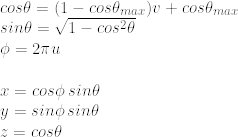Uniform sampling equations for a sphere cap

Where u, v are random numbers in the range of [0,1) and cosθmax is the maximum value of the cosine of theta. That max value is kind of intriguing, how are we going to determine it? It must be related to shininess somehow.  I thought about this a lot, thinking on using derivatives or other tricks, but then looking at current equations I realized that we can pull that value from the importance sampling equations . The cosθ equation looks something like this: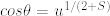This is the cosθ importance sampling equation.

And if we plug a very small value in u, say 1e-10 , we will get a cosθ right where things start to get interesting. Hence the equation we are looking for is:

Where ε is a very small number, 1e-10 for example. Let me show you how cosθmax looks like when different shininess values are used: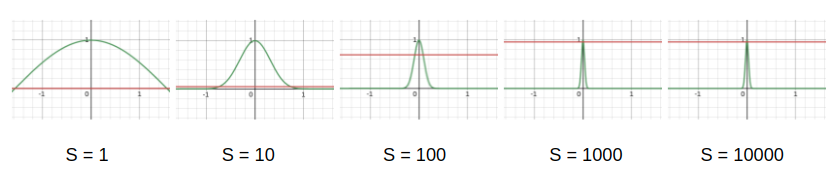cosθmax represented by the red line

Now that we have cosθmax, how does uniform sampling look like when plotted on a sphere ?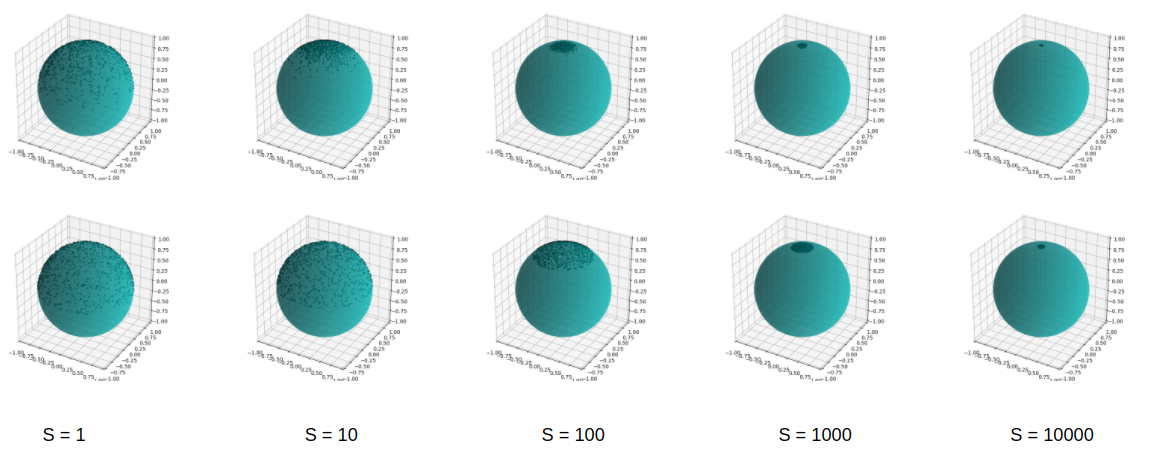Top row: Importance Sampling , Bottom Row: Uniform Sampling

At this point we have almost everything we need to test this idea. We just need a PDF and we are good. The PDF would be 1 / cap area since we are uniform sampling that area and the PDF equation is :

Alright, if there is something I've learned is that providing the Monte Carlo estimator is the right thing to do.

Now we have it all. I plugged these equations in my mighty turtle-tracer to see how things look like. (Note to self : I must finish porting it to Vulkan)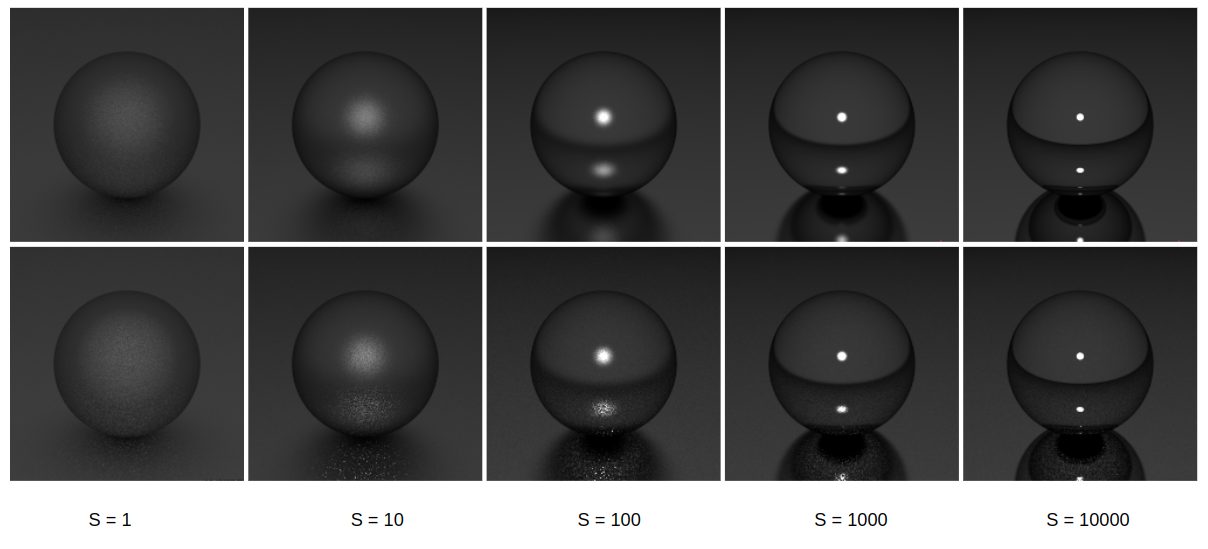Top Row: Importance Sampling, Bottom Row: Uniform Sampling (5000 spp)

So as expected, it's noisy and it takes longer for it to converge, remember, uniform sampling. If we add more samples, it gets there, somewhat.Left: Importance Sampling, Right: Uniform Sampling(30k spp)

So here it is, it works! Kinda....

Just a note, while I was preparing this blog post I noticed that Ray Tracing Gems, Chapter 16 has a method to sample Direction in a cone, which looks a lot like what we just did here, and guess what? I tried those equations and they work as well.